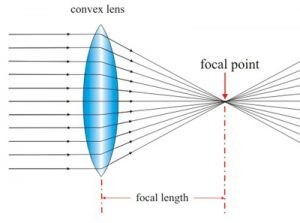convex lens – Rainbow Research Optics

## Understanding a Convex Lens

A lens is a piece of transparent material bound by two surfaces of which at least one is curved. A lens bound by two spherical surfaces bulging outwards is called a bi-convex lens or simply a convex lens. A single piece of glass that curves outward and converges the light incident on it is also called a convex lens. They are both thicker in middle than near the edges. A lens bound by two spherical surfaces curved inwards is called a bi-concave lens or simply a concave lens. It is thinner in the middle than the edges.The central point of a lens is called its optical center. It is usually represented by the letter “o”. For these lenses convex or concave, each surface forms a part of a sphere. The straight line passing through the optical center in the centers of these spheres is called the principle axis.The principle axis is perpendicular to the surfaces of the lens. The effective diameter of a spherical lens is called its aperture. It determines the amount of light that passes through the lens.

To help understand the refraction of light through a convex lens, imagine a convex lens as an arrangement of a number of portions of triangular prisms with a very small glass slab at the center. The base of each prism points towards the principle axis of the lens. We know that a ray of light incident on a prism bends towards its base then emerges out. When a beam of light parallel to the principle axis strikes the lens these rays undergo refraction as they enter the lens and leave it.First, they bend towards the base of the respective prisms and then emerge bending towards the principle axis. Thus, after refraction through the lens they converge to a point on the principle axis on the other side of the lens. This point is called the focal point or principle focus of the lens, and is usually denoted by the letter F. Because a convex lens concentrates the light rays that are incident on it, it is called a converging lens.

The bending of rays is maximal at the top and bottom of the lens. This is because the top and bottom prisms have the greatest angle between the refracting surfaces. As we move towards the principle axis this angle reduces. This causes a decrease in bending of the rays. At the center no bending occurs as the glass bases are parallel. The ray through the optical center emerges un-deviated along its original direction.

The distance from the optical center to the focal point is called the focal length of the lens. According to the sign convention the focal length of the convex lens is positive. Thicker lenses having greater curvature bend the light more and thus have shorter focal length. Thicker convex lenses have more converging power.

If the parallel beams incident on the lens are inclined to the principle axis they converge after refraction to certain points to a plane containing the focal point. The plane passing through all such points perpendicular to the principle axis is called the focal plane of the lens. What if light rays parallel to the principle axis are incident on the other side? The lens will have the same effect on light incident from the other side. Therefore, a convex lens has two focal points usually represented by F1 and F2, one on each side equidistant from the optical center. According to the principle of reversibility of light, a ray of light passing through the focal point of a convex lens emerges on the other side parallel to its principle axis.

Let us now discuss how a convex lens forms images. For different positions of the object a convex lens forms images of varying sizes and nature at different locations. Convex lenses are therefore used in instruments like cameras, telescopes, etc. to form images that we see with our eye, which itself has a lens.

Rainbow Research Optics, Inc. (RROI) offers a variety of custom and stock convex and concave lenses. Send us your quote requests today!